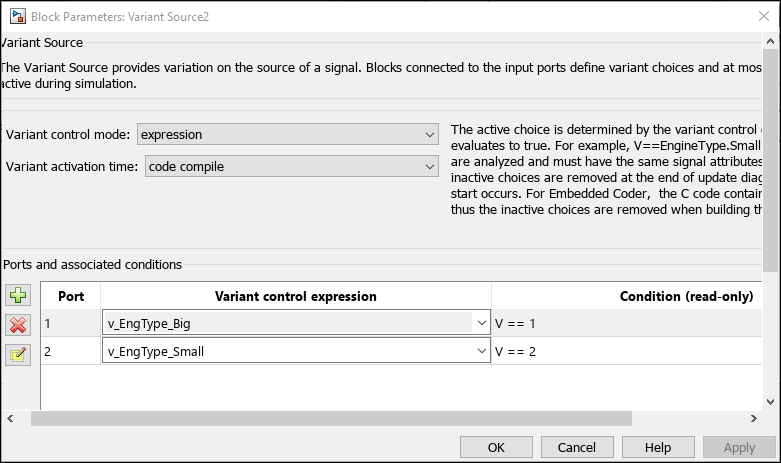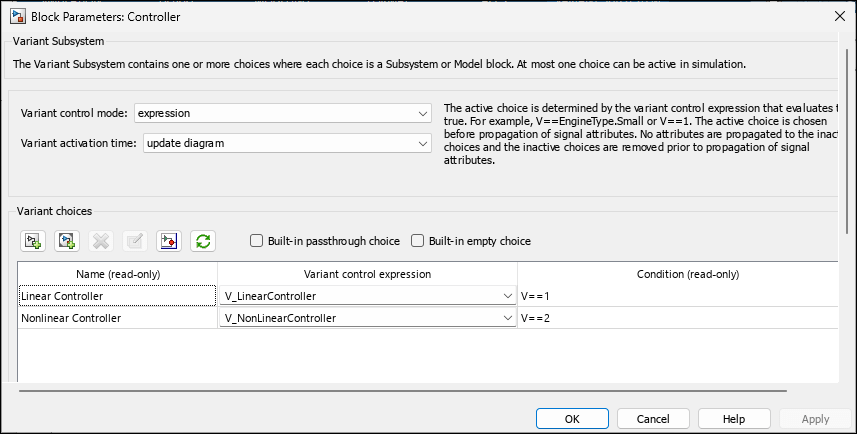Specify conditions that control variant selection

## Description

In a model that contains variant blocks, you must associate each variant choice with a variant control. During simulation, Simulink® evaluates the variant controls of all variant choices and activates the choice that corresponds to the variant control that evaluates to `true`.

You can specify the variant control in the form of a Boolean condition expression, such as `A == 1` and `A==1 && B == 2`. In these expressions, the variant control variables `A` and `B` can be MATLAB® variables, `Simulink.Parameter` objects, or `Simulink.VariantControl` objects. For more information, see Switch Between Choices Using Condition Expressions in Variant Blocks.

Create an object of the `Simulink.Variant` class to represent a Boolean variant condition expression. You can define the object in the base workspace or in a data dictionary and use the object as the variant control for a choice in a variant block. Using `Simulink.Variant` objects lets you reuse common variant conditions across models and improves the readability of variant condition expressions.

In this example, `Simulink.Variant` objects are used as variant controls in a Variant Source block.

```v_EngType_Big = Simulink.Variant('V == 1'); v_EngType_Small = Simulink.Variant('V == 2');```Note

## Creation

### Description

example

````variantControl = Simulink.Variant(conditionExpression)` creates a variant control and sets the Condition property to `conditionExpression`.```

## Properties

expand all

Variant condition expression, specified as a character vector or string. The expression must evaluate to a Boolean value and can contain one or more of these operands and operators.

Operands

• Scalar literal values that represent integer or enumerated values

• Variable names that resolve to one of these types:

• MATLAB variables

• `Simulink.VariantControl` objects

• `Simulink.Parameter` objects with integer, enumerated data type, or scalar literal values

• `Simulink.Variant` objects

Operators

• Parentheses for grouping

• Arithmetic, relational, logical, or bitwise operators

For information on the supported types of operators and operands in a variant condition expression, see Types of Variant Control Variables (Operands) in Variant Blocks and Types of Operators in Variant Blocks for Different Activation Times.

Example: ```'(Fuel==2 || Emission==1) && Ratio==2'```

#### Attributes:

 `GetAccess` `public` `SetAccess` `public`

Data Types: `char` | `string`

## Examples

collapse all

Use MATLAB variables when you want to simulate the model but are not considering code generation.

Create MATLAB variables with scalar literal values.

```Fuel = 3; Emission = 2; Ratio = 3;```

Define conditional expressions using the variables and create variant controls.

```Variant1 = Simulink.Variant('Fuel==3 && Emission==2'); Variant2 = Simulink.Variant('(Fuel==2 || Emission==1) && Ratio==2'); Variant3 = Simulink.Variant('Fuel==3 || Ratio==4');```

To generate preprocessor conditionals for code generation, use `Simulink.Parameter` objects.

Create variant `Simulink.Parameter` objects with scalar literal values.

```Fuel = Simulink.Parameter(3); Emission = Simulink.Parameter(2); Ratio = Simulink.Parameter(3); ```

Specify the custom storage class for these objects as `ImportedDefine` so that the values are specified by an external header file. Other valid values for the custom storage class are `Define` and `CompilerFlag`.

Note

If you generate code with variant activation time set to `startup` , specify the supported custom storage class for the objects. For more information on built-in and custom storage classes supported with `startup` activation time see Storage Classes for Different Variant Activation Times.

```Fuel.CoderInfo.StorageClass = 'Custom'; Fuel.CoderInfo.CustomStorageClass = 'ImportedDefine'; Emission.CoderInfo.StorageClass = 'Custom'; Emission.CoderInfo.CustomStorageClass = 'ImportedDefine'; Ratio.CoderInfo.StorageClass = 'Custom'; Ratio.CoderInfo.CustomStorageClass = 'ImportedDefine'; ```

Define conditional expressions using the variables and create variant controls.

```Variant1 = Simulink.Variant('Fuel==3 && Emission==2'); Variant2 = Simulink.Variant('(Fuel==2 || Emission==1) && Ratio==2'); Variant3 = Simulink.Variant('Fuel==3 || Ratio==4');```

You can associate a variant control variable of type `Simulink.VariantControl` with a variant activation time. For an example, see Simulink.VariantControl Variables for Coherent Switching of Choices in Variant Blocks.

Create `Simulink.VariantControl` objects with scalar literal values.

```Fuel = Simulink.VariantControl('Value',3,'ActivationTime','update diagram'); Emission = Simulink.VariantControl('Value',2,'ActivationTime','update diagram'); Ratio = Simulink.VariantControl('Value',3,'ActivationTime','update diagram'); ```

Define conditional expressions using the variables and create variant controls.

```Variant1 = Simulink.Variant('Fuel==3 && Emission==2'); Variant2 = Simulink.Variant('(Fuel==2 || Emission==1) && Ratio==2'); Variant3 = Simulink.Variant('Fuel==3 || Ratio==4');```

After identifying the variant values that your model requires, you can construct complex variant conditions to control the activation of your variant blocks by defining variant conditions as `Simulink.Variant` objects. `Simulink.Variant` objects enable you to reuse common variant conditions across models and help you encapsulate complex variant condition expressions.

You can specify the whole of a variant condition expression or only the variant control variable inside the `Condition` property of the `Simulink.Variant` object.

Note:

• You can define a variant control variable of type `Simulink.Variant` only in the base workspace or in a data dictionary. Defining variant controls using `Simulink.Variant` objects in the mask or model workspace is not supported. For more information on storage locations for variant control variables, see Storage Locations for Variant Control Variables (Operands) in Variant Blocks.

• Using `Simulink.Variant` within structures is not supported.

Open the `slexVariantSubsystems` model.

`open_system('slexVariantSubsystems');`
```VSS_LINEAR_CONTROLLER = VariantExpression with properties: Condition: 'VSS_MODE==1' ```
```VSS_NONLINEAR_CONTROLLER = VariantExpression with properties: Condition: 'VSS_MODE==2' ```
```VSS_MODE = 2 ```

In the MATLAB® Command Window, define variant control expressions in `Simulink.Variant` objects.

```V_LinearController = Simulink.Variant('V==1'); V_NonLinearController = Simulink.Variant('V==2');```

Specify the `Simulink.Variant` objects as the variant control variables in the block parameters dialog box of the Controller block.

```set_param('slexVariantSubsystems/Controller/Linear Controller', 'VariantControl', 'V_LinearController') set_param('slexVariantSubsystems/Controller/Nonlinear Controller', 'VariantControl', 'V_NonLinearController')```

Open the Block Parameters dialog box for the `Controller` block in the model. The Condition column of the Variant Choices table automatically shows the Boolean conditions that the `Simulink.Variant` objects represent.Set the value of variant control variable `V` as `1` and simulate the model.

```V = 1; sim('slexVariantSubsystems');```

During simulation, the `Linear Controller` block becomes active. Double-click the `Controller` block to see the active choice. Using this approach, you can develop complex variant condition expressions that are reusable.

## Version History

Introduced before R2006a